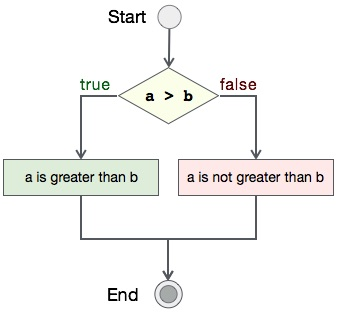# Program to write file in C

## Algorithm

Let's first see what should be the step-by-step procedure to compare two integers−

```START

Step 1 → Take two integer variables, say A & B

Step 2 → Assign values to variables

Step 3 → Compare variables if A is greater than B

Step 4 → If true print A is greater than B

Step 5 → If false print A is not greater than B

STOP
```

## Flow Diagram

We can draw a flow diagram for this program as given below −## Pseudocode

Let's now see the pseudocode of this algorithm −

```procedure compare(A, B)

IF A is greater than B
DISPLAY "A is greater than B"
ELSE
DISPLAY "A is not greater than B"
END IF

end procedure
```

## Implementation

Now, we shall see the actual implementation of the program −

```#include <stdio.h>

int main()
{
FILE *fp;
char *filename = "sample.txt";
char *content = "Hey there!  You've successfully created a file with content in c programming language.";

/*  open for writing */
fp = fopen(filename, "w");

if( fp == NULL )
{
printf("%s: failed to open. \n", filename);
return -1;
}
else
{
printf("%s: opened in write mode.\n", filename);
}

/* Write content to file */
fprintf(fp, "%s\n", content);

if( !fclose(fp) )
printf("%s: closed successfully.\n", filename);

return 0;
}
```

## Output

Output of this program should be −

```sample.txt: opened in write mode.
sample.txt: closed successfully.
```